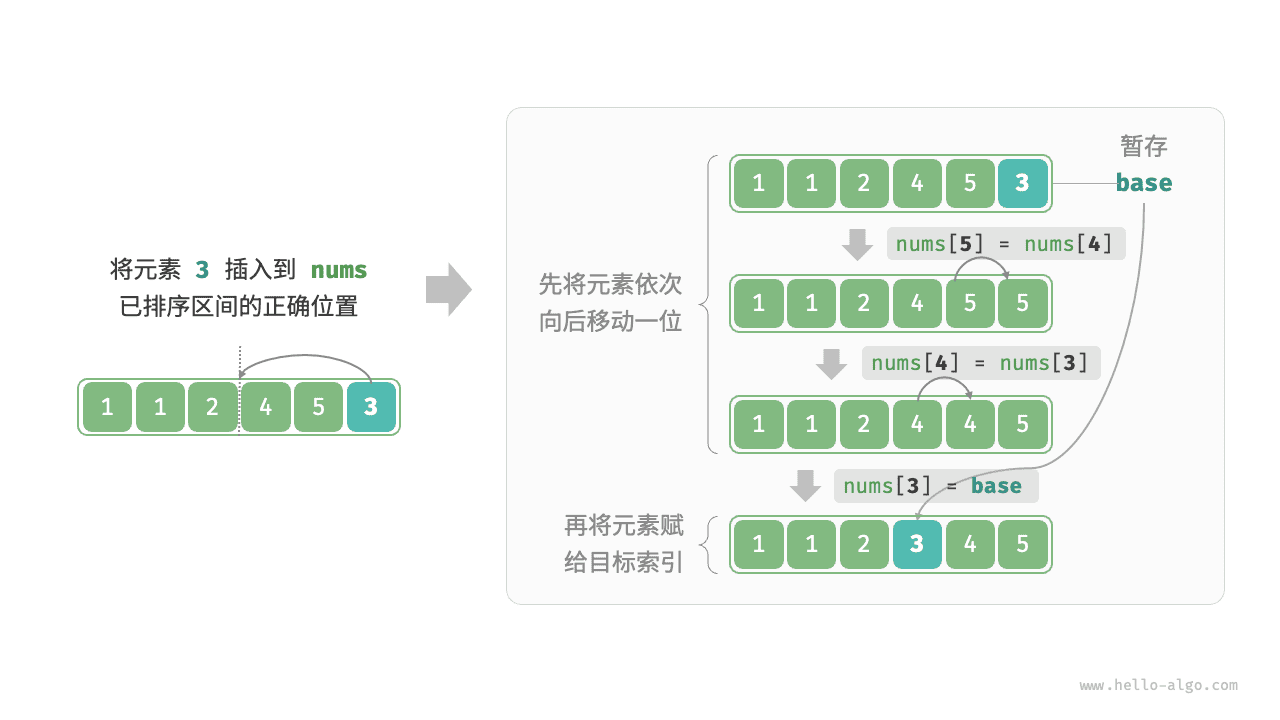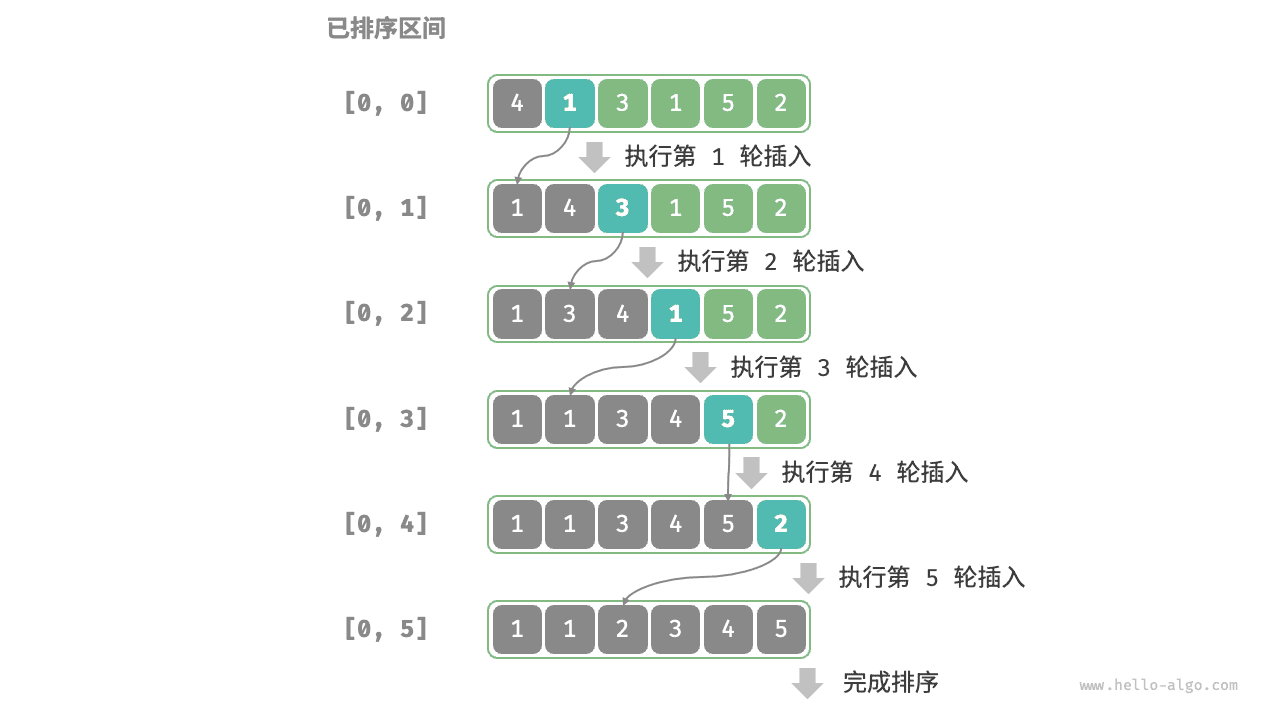# 11.3.   插入排序¶

「插入排序 Insertion Sort」是一种基于 数组插入操作 的排序算法。

「插入操作」原理：选定某个待排序元素为基准数 base，将 base 与其左侧已排序区间元素依次对比大小，并插入到正确位置。Fig. 单次插入操作

## 11.3.1.   算法流程¶

1. 先选取数组的 第 2 个元素base ，执行插入操作后，数组前 2 个元素已完成排序
2. 选取 第 3 个元素base ，执行插入操作后，数组前 3 个元素已完成排序
3. 以此类推……最后一轮选取 数组尾元素base ，执行插入操作后，所有元素已完成排序Fig. 插入排序流程

insertion_sort.java
/* 插入排序 */
void insertionSort(int[] nums) {
// 外循环：base = nums, nums, ..., nums[n-1]
for (int i = 1; i < nums.length; i++) {
int base = nums[i], j = i - 1;
// 内循环：将 base 插入到左边的正确位置
while (j >= 0 && nums[j] > base) {
nums[j + 1] = nums[j];  // 1. 将 nums[j] 向右移动一位
j--;
}
nums[j + 1] = base;         // 2. 将 base 赋值到正确位置
}
}

insertion_sort.cpp
/* 插入排序 */
void insertionSort(vector<int>& nums) {
// 外循环：base = nums, nums, ..., nums[n-1]
for (int i = 1; i < nums.size(); i++) {
int base = nums[i], j = i - 1;
// 内循环：将 base 插入到左边的正确位置
while (j >= 0 && nums[j] > base) {
nums[j + 1] = nums[j];  // 1. 将 nums[j] 向右移动一位
j--;
}
nums[j + 1] = base;         // 2. 将 base 赋值到正确位置
}
}

insertion_sort.py
def insertion_sort(nums: list[int]) -> None:
""" 插入排序 """
# 外循环：base = nums, nums, ..., nums[n-1]
for i in range(1, len(nums)):
base: int = nums[i]
j: int = i - 1
# 内循环：将 base 插入到左边的正确位置
while j >= 0 and nums[j] > base:
nums[j + 1] = nums[j]  # 1. 将 nums[j] 向右移动一位
j -= 1
nums[j + 1] = base         # 2. 将 base 赋值到正确位置

insertion_sort.go
/* 插入排序 */
func insertionSort(nums []int) {
// 外循环：待排序元素数量为 n-1, n-2, ..., 1
for i := 1; i < len(nums); i++ {
base := nums[i]
j := i - 1
// 内循环：将 base 插入到左边的正确位置
for j >= 0 && nums[j] > base {
nums[j+1] = nums[j] // 1. 将 nums[j] 向右移动一位
j--
}
nums[j+1] = base // 2. 将 base 赋值到正确位置
}
}

insertion_sort.js
/* 插入排序 */
function insertionSort(nums) {
// 外循环：base = nums, nums, ..., nums[n-1]
for (let i = 1; i < nums.length; i++) {
let base = nums[i], j = i - 1;
// 内循环：将 base 插入到左边的正确位置
while (j >= 0 && nums[j] > base) {
nums[j + 1] = nums[j];  // 1. 将 nums[j] 向右移动一位
j--;
}
nums[j + 1] = base;         // 2. 将 base 赋值到正确位置
}
}

insertion_sort.ts
/* 插入排序 */
function insertionSort(nums: number[]): void {
// 外循环：base = nums, nums, ..., nums[n-1]
for (let i = 1; i < nums.length; i++) {
const base = nums[i];
let j = i - 1;
// 内循环：将 base 插入到左边的正确位置
while (j >= 0 && nums[j] > base) {
nums[j + 1] = nums[j]; // 1. 将 nums[j] 向右移动一位
j--;
}
nums[j + 1] = base; // 2. 将 base 赋值到正确位置
}
}

insertion_sort.c
[class]{}-[func]{insertionSort}

insertion_sort.cs
/* 插入排序 */
void insertionSort(int[] nums)
{
// 外循环：base = nums, nums, ..., nums[n-1]
for (int i = 1; i < nums.Length; i++)
{
int bas = nums[i], j = i - 1;
// 内循环：将 base 插入到左边的正确位置
while (j >= 0 && nums[j] > bas)
{
nums[j + 1] = nums[j]; // 1. 将 nums[j] 向右移动一位
j--;
}
nums[j + 1] = bas;         // 2. 将 base 赋值到正确位置
}
}

insertion_sort.swift
/* 插入排序 */
func insertionSort(nums: inout [Int]) {
// 外循环：base = nums, nums, ..., nums[n-1]
for i in stride(from: 1, to: nums.count, by: 1) {
let base = nums[i]
var j = i - 1
// 内循环：将 base 插入到左边的正确位置
while j >= 0, nums[j] > base {
nums[j + 1] = nums[j] // 1. 将 nums[j] 向右移动一位
j -= 1
}
nums[j + 1] = base // 2. 将 base 赋值到正确位置
}
}

insertion_sort.zig
// 插入排序
fn insertionSort(nums: []i32) void {
// 外循环：base = nums, nums, ..., nums[n-1]
var i: usize = 1;
while (i < nums.len) : (i += 1) {
var base = nums[i];
var j: usize = i;
// 内循环：将 base 插入到左边的正确位置
while (j >= 1 and nums[j - 1] > base) : (j -= 1) {
nums[j] = nums[j - 1];  // 1. 将 nums[j] 向右移动一位
}
nums[j] = base;             // 2. 将 base 赋值到正确位置
}
}


## 11.3.3.   插入排序优势¶

• 冒泡操作基于 元素交换 实现，需要借助一个临时变量实现，共 3 个单元操作；
• 插入操作基于 元素赋值 实现，只需 1 个单元操作；

• 对于 长数组，采用基于分治的排序算法，例如「快速排序」，时间复杂度为 $$O(n \log n)$$
• 对于 短数组，直接使用「插入排序」，时间复杂度为 $$O(n^2)$$